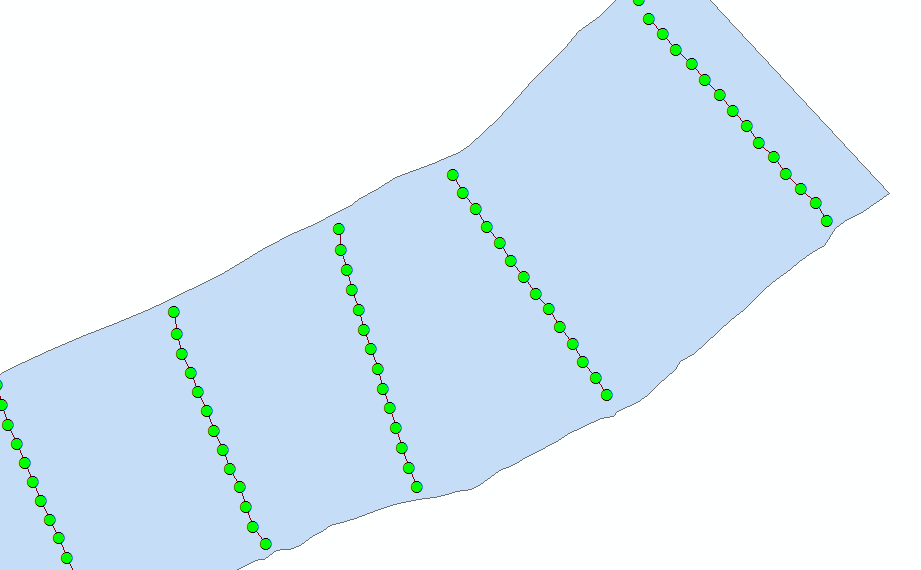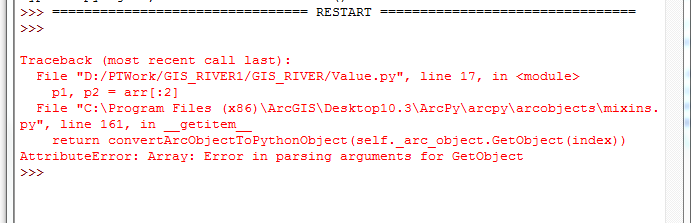# Draw a Line to the nearest Polygon.

3031
9
04-09-2017 07:55 AMOccasional Contributor II

Hi,

I've some set of points which I'm joining to make a line. Refer the Picture below.The problem I'm facing is there are no points(elevation points) on the polygon (River Bank). Is there any way of extending the line to the polygon?

Tags (3)
9 RepliesbyMVP Legendary Contributor

Extend line comes to mind, but your polygon may have to be converted to a polyline.  There are other coding examples that turn up using a web search if you don't have the appropriate licensebyMVP Esteemed Contributor

My first thought was Extend line, as Dan already mentioned, but then I remembered that it operates within a single feature class, which would involve creating a new, intermediate data set that includes the boundaries of the polygons.  Even then, there are questions about how close the lines are to the boundary versus other lines, and what exactly would be extended to what.

Another approach is to loop through all of the polygons, river banks in this example, select all of the lines that are within a given polygon, and extend those lines using one of a variety of techniques to match up with each containing polygon.  This sample code worked for a basic example I came up with:

``````import math

dist = 100 # distance, in units of projection, to extend the line on each end

fc_line = # path to feature class containing lines
fc_line = arcpy.MakeFeatureLayer_management(fc_line, "fc_line")

fc_poly = # path to feature class containing polygon
fc_poly = arcpy.MakeFeatureLayer_management(fc_poly, "fc_poly")

with arcpy.da.SearchCursor(fc_poly, "SHAPE@") as scur:
for poly, in scur:
boundary = poly.boundary()
arcpy.SelectLayerByLocation_management(fc_line, "WITHIN", poly)
with arcpy.da.UpdateCursor(fc_line, "SHAPE@") as ucur:
for line, in ucur:
arr, = line.getPart()
SR = line.spatialReference

p1, p2 = arr[:2]
angle = math.atan2(p2.Y - p1.Y, p2.X - p1.X)
p = arcpy.Point(p1.X - dist * math.cos(angle),
p1.Y - dist * math.sin(angle))
arr.insert(0, p)

pn1, pn = arr[-2:]
angle = math.atan2(pn.Y - pn1.Y, pn.X - pn1.X)
p = arcpy.Point(pn.X + dist * math.cos(angle),
pn.Y + dist * math.sin(angle))
arr.append(p)

line = arcpy.Polyline(arr, SR)
line = line.cut(boundary)
ucur.updateRow([line])‍‍‍‍‍‍‍‍‍‍‍‍‍‍‍‍‍‍‍‍‍‍‍‍‍‍‍‍‍‍‍‍‍‍‍‍‍‍‍‍‍‍‍‍‍‍‍‍‍‍‍‍‍‍‍‍‍‍‍‍‍‍‍‍‍‍‍‍``````

Since I don't know the exact structure of your feature classes, I am not sure whether my code would work without some modification.

As Dan mentions, there are numerous ways to extend the line.  One simple approach is to go from the end points of the line to the closest points on the polygon.  I am not a fan of that approach because it could create an unnatural kink/turn in the line depending on the angle the line is relative to the polygon.  A much fancier approach is to use NumPy and SciPy to do form fitting of the lines and then extrapolate using robust math equations.

The code above splits the difference, in a way, in that it linearly extrapolates the ends from the last two points on each end.  Instead of trying to match up the new line exactly, I actually extend the line farther than the polygon and use the cut() method of an ArcPy Geometry object to trim the line back to the boundary of the polygon.

Also, the code above assumes the data is stored within a projection because I am using simple Cartesian math to measure angles and extend lines instead of using geodesic math.byMVP Frequent Contributor

Another approach would be to convert the polygon to a polyline then use

with the nearest option. Include the proximity info, this gives you the coordinate of the near point.Occasional Contributor II

Getting the following error when I'm building the tool:My code for your reference :

import arcpy,math
fc_line = r'd:/PTWork/GIS_RIVER/RiverBank.shp'
fc_line = arcpy.MakeFeatureLayer_management(fc_line, "fc_line")

fc_poly = r'd:/PTWork/GIS_RIVER/RB_23.shp'
fc_poly = arcpy.MakeFeatureLayer_management(fc_poly, "fc_poly")

with arcpy.da.SearchCursor(fc_poly, "SHAPE@") as scur:
for poly, in scur:
boundary = poly.boundary()
arcpy.SelectLayerByLocation_management(fc_line, "WITHIN", poly)
lst_value =[]
with arcpy.da.UpdateCursor(fc_line, "SHAPE@") as ucur:
for line, in ucur:
arr, = line.getPart()
SR = line.spatialReference
p1, p2 = arr[:2]
angle = math.atan2(p2.Y - p1.Y, p2.X - p1.X)
p = arcpy.Point(p1.X - dist * math.cos(angle),
p1.Y - dist * math.sin(angle))
arr.insert(0, p)

pn1, pn = arr[-2:]
angle = math.atan2(pn.Y - pn1.Y, pn.X - pn1.X)
p = arcpy.Point(pn.X + dist * math.cos(angle),
pn.Y + dist * math.sin(angle))
arr.append(p)

line = arcpy.Polyline(arr, SR)
line = line.cut(boundary)
ucur.updateRow([line])byMVP Legendary Contributor

How to Format your code... That link will provide you with instructions, otherwise what you have posted is of little use to decipher potential errors.Occasional Contributor II

Thanks !Occasional Contributor II

import arcpy
import math
import os
import numpy as np
from numpy import trapz
arcpy.env.overwriteOutput = True

fc = r'D:/PTWork/GIS_RIVER/23_elevation.shp'
fld_memo = 'TxtMemo'
fc_river = r'D:/PTWork/GIS_RIVER/RB_23.shp'
fc_out = r'D:/PTWork/GIS_RIVER/RiverBank1.shp'
fld_id = 'LineID'
id_format = 'CS-{}'
tolerance = 350
dist = 100 # distance, in units of projection, to extend the line on each end

flds = ('OID@', 'SHAPE@', fld_memo)
lst_data = [[r, r, float(r)] for r in arcpy.da.SearchCursor(fc, flds)]

# create output featureclass
sr = arcpy.Describe(fc).spatialReference
ws, fc_name = os.path.split(fc_out)
arcpy.CreateFeatureclass_management(ws, fc_name, "POLYLINE", None, None, "ENABLED", sr)
arcpy.AddField_management(fc_out, fld_id, "TEXT", None, None, 25)

# insert cursor
flds = ('SHAPE@', fld_id)
cnt_id = 0
with arcpy.da.InsertCursor(fc_out, flds) as curs:
lst_lines = []
lst_pnts = []
for i in range(1, len(lst_data)):
print("i:{}".format(i))
lst_cur = lst_data
lst_prev = lst_data[i-1]
pntg_cur = lst_cur
pntg_prev = lst_prev
dist = pntg_prev.distanceTo(pntg_cur)

if dist <= tolerance:
pnt = pntg_cur.firstPoint
z = lst_cur
pnt.Z = z
lst_pnts.append(pnt)
else:
print(" - create and store line and add point to new list...")
# create and store line and add point to new list
if len(lst_pnts) > 1:
polyline = arcpy.Polyline(arcpy.Array(lst_pnts), sr, True, False)
lst_lines.append(polyline)
cnt_id += 1
line_id = id_format.format(cnt_id)
fc_line = arcpy.MakeFeatureLayer_management(polyline, "fc_line")
fc_poly = arcpy.MakeFeatureLayer_management(fc_river, "fc_poly")
with arcpy.da.SearchCursor(fc_poly, "SHAPE@") as scur:
for poly, in scur:
boundary = poly.boundary()
arcpy.SelectLayerByLocation_management(fc_line, "WITHIN", poly)
with arcpy.da.SearchCursor(fc_line, "SHAPE@") as ucur:
for line, in ucur:
arr, = line.getPart()
SR = line.spatialReference
p1, p2 = arr[:2]
angle = math.atan2(p2.Y - p1.Y, p2.X - p1.X)
p = arcpy.Point(p1.X - dist * math.cos(angle),
p1.Y - dist * math.sin(angle))
arr.insert(0, p)

pn1, pn = arr[-2:]
angle = math.atan2(pn.Y - pn1.Y, pn.X - pn1.X)
p = arcpy.Point(pn.X + dist * math.cos(angle),
pn.Y + dist * math.sin(angle))
arr.append(p)

line = arcpy.Polyline(arr, SR)
line = line.cut(boundary)

curs.insertRow((line, line_id))
else:
# not enough points to create line
pass
pnt = pntg_cur.firstPoint
z = lst_cur
pnt.Z = z
lst_pnts = [pnt]Occasional Contributor II

import arcpy
import math
import os
import numpy as np
from numpy import trapz
arcpy.env.overwriteOutput = True

fc = r'D:/PTWork/GIS_RIVER/23_elevation.shp'
fld_memo = 'TxtMemo'
fc_river = r'D:/PTWork/GIS_RIVER/RB_23.shp'
fc_out = r'D:/PTWork/GIS_RIVER/RiverBank1.shp'
fld_id = 'LineID'
id_format = 'CS-{}'
tolerance = 350
dist = 100 # distance, in units of projection, to extend the line on each end

flds = ('OID@', 'SHAPE@', fld_memo)
lst_data = [[r, r, float(r)] for r in arcpy.da.SearchCursor(fc, flds)]

# create output featureclass
sr = arcpy.Describe(fc).spatialReference
ws, fc_name = os.path.split(fc_out)
arcpy.CreateFeatureclass_management(ws, fc_name, "POLYLINE", None, None, "ENABLED", sr)
arcpy.AddField_management(fc_out, fld_id, "TEXT", None, None, 25)

# insert cursor
flds = ('SHAPE@', fld_id)
cnt_id = 0
with arcpy.da.InsertCursor(fc_out, flds) as curs:
lst_lines = []
lst_pnts = []
for i in range(1, len(lst_data)):
print("i:{}".format(i))
lst_cur = lst_data
lst_prev = lst_data[i-1]
pntg_cur = lst_cur
pntg_prev = lst_prev
dist = pntg_prev.distanceTo(pntg_cur)

if dist <= tolerance:
pnt = pntg_cur.firstPoint
z = lst_cur
pnt.Z = z
lst_pnts.append(pnt)
else:
print(" - create and store line and add point to new list...")
# create and store line and add point to new list
if len(lst_pnts) > 1:
polyline = arcpy.Polyline(arcpy.Array(lst_pnts), sr, True, False)
lst_lines.append(polyline)
cnt_id += 1
line_id = id_format.format(cnt_id)
fc_line = arcpy.MakeFeatureLayer_management(polyline, "fc_line")
fc_poly = arcpy.MakeFeatureLayer_management(fc_river, "fc_poly")
with arcpy.da.SearchCursor(fc_poly, "SHAPE@") as scur:
for poly, in scur:
boundary = poly.boundary()
arcpy.SelectLayerByLocation_management(fc_line, "WITHIN", poly)
with arcpy.da.SearchCursor(fc_line, "SHAPE@") as ucur:
for line, in ucur:
arr, = line.getPart()
SR = line.spatialReference
p1, p2 = arr[:2]
angle = math.atan2(p2.Y - p1.Y, p2.X - p1.X)
p = arcpy.Point(p1.X - dist * math.cos(angle),
p1.Y - dist * math.sin(angle))
arr.insert(0, p)

pn1, pn = arr[-2:]
angle = math.atan2(pn.Y - pn1.Y, pn.X - pn1.X)
p = arcpy.Point(pn.X + dist * math.cos(angle),
pn.Y + dist * math.sin(angle))
arr.append(p)

line = arcpy.Polyline(arr, SR)
line = line.cut(boundary)

curs.insertRow((line, line_id))
else:
# not enough points to create line
pass
pnt = pntg_cur.firstPoint
z = lst_cur
pnt.Z = z
lst_pnts = [pnt]byMVP Legendary Contributor

still lacking proper formatting ie indentation

in any event, p1, p2 = a[:2] means it is expecting 2 points... it will fail if it doesn't get 2